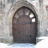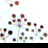# expression for TF/FT_MINUTES

3630
3
11-05-2013 12:11 AMNew Contributor
Hi
You know there is Shape_Length field with the length of line features, which is calculated automatically.
I basically want to make my FT/TF_Minutes fields doing smth like the same - i ve got also Speedlimit field with km/hour, so the question is if it is pissible to calculate FT/TF_Minutes automatically everytime i change Speedlimit or Create new obj and so on.
The expressions seems to look like this:
FT_MINUTES
[SHAPE_Length] * 60 / ([Spdlimit_F] * 1000)
TF_MINUTES
[SHAPE_Length] * 60 / ([Spdlimit_B] * 1000
but i have no idea where can i put it. First i thought about Default Value in Feature Class properties, but it didnt work.
So i started my topic here cause its related to Network Analyst...

Thanks
Tags (2)
3 RepliesNew Contributor III
Hi
You know there is Shape_Length field with the length of line features, which is calculated automatically.
I basically want to make my FT/TF_Minutes fields doing smth like the same - i ve got also Speedlimit field with km/hour, so the question is if it is pissible to calculate FT/TF_Minutes   automatically everytime i change Speedlimit or Create new obj and so on.
The expressions seems to look like this:
FT_MINUTES
[SHAPE_Length] * 60 / ([Spdlimit_F] * 1000)
TF_MINUTES
[SHAPE_Length] * 60 / ([Spdlimit_B] * 1000

but i have no idea where can i put it. First i thought about Default Value in Feature Class properties, but it didnt work.
So i started my topic here cause its related to Network Analyst...

Thanks

If you want the calculations to be part of the feature (or shapefile), you can create the fields the use the field calculator to fill them in. If you just want it in the network dataset, you add them when you get to the network attributes. Look at #23 and 24 in the Network Analyst tutorial, Exercise 1: http://resources.arcgis.com/en/help/main/10.2/index.html#//00470000005t000000).

I don't know if the calculations/evaluators are dynamic - you might need to rebuild the network (one step with the toolbox) or recalculate the fields (if you make them part of the feature.)New Contributor
If you want the calculations to be part of the feature (or shapefile), you can create the fields the use the field calculator to fill them in.  If you just want it in the network dataset, you add them when you get to the network attributes.  Look at #23 and 24 in the Network Analyst tutorial, Exercise 1:  http://resources.arcgis.com/en/help/main/10.2/index.html#//00470000005t000000).

I don't know if the calculations/evaluators are dynamic - you might need to rebuild the network (one step with the toolbox) or recalculate the fields (if you make them part of the feature.)

Well, if i choose the first way then i ll have to recalculate fields every time i change speed limit or length. It means opening field calc, typing expressions for both of my fields, etc. Ok, done with it)
If i just want to have Total time in Directions Window in Network Analyst(NA), and info about the time is recieved from fields TF_MINUTES and FT_MINUTES as the NA recodnizes them, how can i get this value (i mean Total time) without TF_MINUTES and FT_MINUTES because i cant change their values just using NA? If its not possible it brings me to the first way.
But If there is a script or expression to calculate Total time using just Shape_Length and Speedlimit that ll be the solution.New Contributor
I did a similar project with FT_Minutes and TF_Minutes, but the values didn't change automatically- everytime I had to use the formula in Field Calculator. Shape_Length field, however, changes automatically.

Koushik Paul